# Array in JavaScript

## Introduction

In the previous chapter, we learned about Math Object and its uses in JavaScript.

In this chapter, we will learn about Arrays, its syntaxes and how array used in the programs of JavaScript.

## Array in JavaScript

Array is a collection of data items stored under a single name. Array provides a framework for announcing and accessing multiple data Objects should have only one identifier, thereby simplifying the task of data management.

We use an array when we have to deal with multiple data items. Arrays are a special type of object in JavaScript. If we want to verify that array is an object that we can check it through "typeof" operator.

### Array syntax in JavaScript

1. var array_name= ["one", "two", "three", "four"];
The complete program of Array in JavaScript is listed below.
1. <!DOCTYPE html>
2. <html>
3.     <body>
4.
5.     <h2>First Array Example Program</h2>
6.     <p>First paragraph.</p>
7.     <p>JavaScript code starts here....</p>
8.
9.     <script>
10.         var stu = ["Rahul""Rohit""Rohan"];
11.         document.write(stu);
12.     </script>
13.
14.     <p>JavaScript code ends here......</p>
15.     </body>
16. </html>
The above program generates the following output.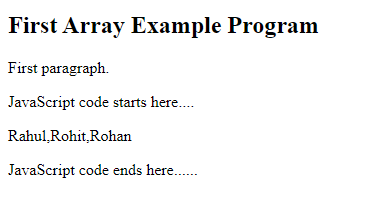Explanation

In the above array example program, we use JavaScript code inside the HTML code. So, first, we print a heading into the <h2> tag "First Array Example Program". Then we print two-paragraphs by using <p> tag, As "First Paragraph" and "JavaScript code starts here....". After that we write JavaScript code as <script> tag. Inside <script> tag, first we define an array named stu. In the next line, we access the array elements bt "document.write(stu);" line which prints array elements separated by commas as the output. In the last line, we print a paragraph" JavaScript code ends here....".

## How to access the array elements?

Array elements can be assessed through the index of the element. Array index starts with 0. For example, if we have 5 elements in an array. So the index will be like , , , , . we can access the full array then we will use the array name for this. But if we want to access any specific element of the array then, we will use the index number like if we want to access the third element of the array we will use  index of the array.

As we have seen in the above array example program we access the full array through the array name stu. Through the statement "document.write(stu);" we print the full array.

The complete program for showing the process of accessing the array elements is listed below.
1. <!DOCTYPE html>
2. <html>
3.     <body>
4.     <h2>How to access array elements</h2>
5.
6.     <script>
8.         document.write(ele);
9.     </script>
10.
11.       </body>
12. </html>
The above program generates the following output.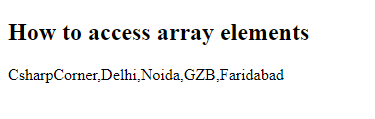Explanation

In the above example program, we define an array named "ele" and access that by the statement "document.write(ele); ". It returns the elements of the array as the output of the program.

### Access elements by index

In array elements accessed by the index number like ele for the first element, ele for the second element, etc.

The complete program for showing how to access array elements by the index number.
1. <!DOCTYPE html>
2. <html>
3.     <body>
4.     <h2>How to access array elements by index</h2>
5.
6.     <script>
8.         document.write(ele);
9.     </script>
10.
11.       </body>
12. </html>
The above program generates the following program.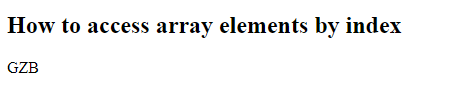Explanation

In the above program, we access the forth the element of the array list as the statement "document.write(ele);" which returns "GZB" as the element.

### Replace the Array elements in JavaScript

The complete program for showing how to replace the array elements in JavaScript.
1. <!DOCTYPE html>
2. <html>
3.     <body>
4.     <h2>How to access array elements by index</h2>
5.
6.     <script>
8.         document.write(ele);
9.         ele= "Panipat";
10.         document.write(ele);
11.     </script>
12.         <p> Value changed GZB to Panipat</p>
13.     </body>
14. </html>
The following program generates the following output.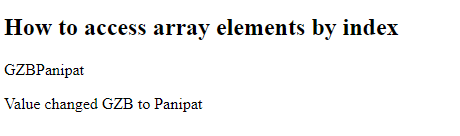Explanation

In the above program, first, we print the fourth element of the array from the list that is "GZB", after that we change the value of "GZB" to "Panipat".

## Array Methods in JavaScript

Array in JavaScript contains various methods for performing basic operations. The various array methods are as follows:

### concat() Method of Array

The concat() method is used to merge two or more arrays. It does not change the existing arrays but returns a new array as the output.

The complete program of concat() method of Array in JavaScript is listed below.
1. <!DOCTYPE html>
2. <html>
3.     <body>
4.     <h3>Example program of concat method</h3>
5.
6.     <script>
8.         var arr2 = ["Delhi""Haryana""UP"];
9.         var arr3 = ["Rohan""Rohit"];
10.         var arr = arr1.concat(arr2,arr3);
11.
12.         document.write(arr);
13.     </script>
14.     </body>
15. </html>
The above program generates the following output.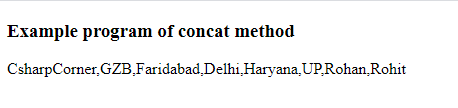Explanation

In the above example program, first, we print a heading through <h3> tag that prints "Example program of concat method". After that JavaScript code starts, in this we create three arrays and in the other forth array, we call concat method. Through the statement "document.write" statement we print the result of the program.

### copyWithin() method of Array

copyWithin() method is used to copy the elements of the array from one place to another. This method overwrites the original value of the element form the other element.

The complete program of copyWithin() method of the array in JavaScript is listed below.
1. <!DOCTYPE html>
2. <html>
3.     <body>
4.         <button onclick="arrayCopyWithin()">Click</button>
5.         <p id="pDemo"></p>
6.         <script>
7.             var colors=["Red","Green","Blue"];
8.             function arrayCopyWithin() {
9.                 document.getElementById("pDemo").innerHTML = colors.copyWithin(1,0);
10.             }
11.         </script>
12.     </body>
13. </html>
14. </html>
The above program generates the following output.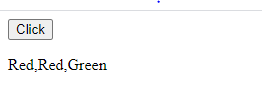Explanation

In the above program, first, we build a button named "click". After that JavaScript code starts. In JavaScript code, we create an array, and inside that method we use the copyWithin method. In the output, We found the button on the screen that shows click. When we click the button the result shows as the output image.

### fill() method of Array

fill method in JavaScript array elements will override with a specific element. It works as a static value.

The complete program of fill() method in JavaScript is listed below.
1. <!DOCTYPE html>
2. <html>
3.     <body>
4.     <html>
5.     <body>
6.         <button onclick="fillTheArray()">Click</button>
7.         <p id="pDemo"></p>
8.         <script>
9.             var cities=["Delhi","GZB","Noida"];
10.             function fillTheArray() {
11.                 document.getElementById("pDemo").innerHTML = friends.fill("UP");
12.             }
13.         </script>
14.     </body>
15. </html>
The above program generates the following output.Explanation

In the above program, first, we build a button with the name "click". After that JavaScript code starts. In JavaScript code, we create an array named cities with three elements, inside a user-defined array we call fill() method. In the output, we found a button "click" when we click the button we get the result shown in the output image.

## Length property of Array in JavaScript

The length property of the array is used to get the length of the array in JavaScript. It returns the number of the array as the output. The value is an unsigned, 32-bit integer and is always numerically bigger than the array's highest number.

The complete program for showing the use of length property of the array in JavaScript is listed below.
1. <!DOCTYPE html>
2. <html>
3.     <body>
4.     <html>
5.     <body>
6.         <h2>Length Property Example Program</h2>
7.
8.         <script>
9.             var cities=["Delhi","GZB","Noida""UP"];
10.                 document.write(cities.length);
11.         </script>
12.
13.     </body>
14. </html>
The above program generates the following output.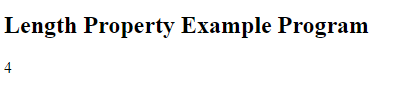Explanation

In the above program, first, we print a heading in <h2> tag. After that JavaScript code starts, here we create an array named cities. As the output, we get the number of the elements of the array as shown in the output image.

## Summary

In this chapter, we learned about the array, how to access the arrays and its methods in JavaScript with the example programs.
Next » Document Object Model in JavaScript - Part One